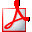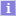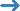Uniwersytet Warszawski - Centralny System Uwierzytelniania Nie jesteś zalogowany | zaloguj sięDrukuj sylabus

# Topological Field Theory

## Informacje ogólne

 Kod przedmiotu: 1100-3`TFT Kod Erasmus / ISCED: 13.204/ (0533) FizykaNazwa przedmiotu: Topological Field Theory Jednostka: Wydział Fizyki Grupy: Fizyka, II stopień; przedmioty do wyboru Fizyka; przedmioty prowadzone w języku angielskim Przedmioty do wyboru dla doktorantów; Punkty ECTS i inne: (brak)zobacz reguły punktacji Język prowadzenia: angielski Rodzaj przedmiotu: monograficzne Założenia (opisowo): (tylko po angielsku) The lecture should be accessible for second and third year students, as well as MSc students; it should also be of interests to PhD students and anyone interested in various relations between physics and mathematics. The knowledge of mathematical analysis and classical mechanics is required; the acquaintance with (at least) basics of quantum mechanics and classical field theory will be of advantage. Skrócony opis: (tylko po angielsku) In this lecture the following topics will be discussed: - various constructions of topological field theories: Schwarz-type, Witten-type - basics of supersymmetry, supersymmetric quantum mechanics, Witten index - localization, homological theories, topological twist - Chern-Simons theory, elements of knot theory - ‘t Hooft topological (“large N”) expansion for field theories and matrix models - axiomatic formulation of topological field theories - modern applications Pełny opis: (tylko po angielsku) Topological field theories provide one of the simplest examples of quantum field theories, and constitute a fascinating research area at the border of physics and mathematics. They are often exactly solvable and illustrate various fundamental phenomena, such as a role of topologically non-trivial solutions, non-perturbative effects, supersymmetry, etc. In physics, topological field theories find applications in condensed matter theory, quantum information, high energy, or string theory. From mathematical viewpoint they provide an important ground for rigorous formulation of quantum field theory, and at the same time are related to knot theory, low-dimensional topology, moduli spaces in algebraic geometry, etc. Several distinguished mathematicians and mathematical physicists, such as Donaldson, Jones, Witten, and Kontsevich, received Fields medals for their work related to topological field theory. In this lecture the following topics will be discussed: - various constructions of topological field theories: Schwarz-type, Witten-type - basics of supersymmetry, supersymmetric quantum mechanics, Witten index - localization, homological theories, topological twist - Chern-Simons theory, elements of knot theory - ‘t Hooft topological (“large N”) expansion for field theories and matrix models - axiomatic formulation of topological field theories - modern applications The lecture should be accessible for second and third year students, as well as MSc students; it should also be of interests to PhD students and anyone interested in various relations between physics and mathematics. The knowledge of mathematical analysis and classical mechanics is required; the acquaintance with (at least) basics of quantum mechanics and classical field theory will be of advantage. Literatura: (tylko po angielsku) Some (parts of) original papers: - E. Witten, "Supersymmetry and Morse theory", J. Diff. Geom. 17 (1982) 4, 661 - L. Alvarez-Gaume, "Supersymmetry and the Atiyah-Singer index theorem", Commun. Math. Phys. 90 (1983) 161 - M. Atiyah, "New invariants of 3- and 4-dimensional manifolds", Proc. Symp. Pure Math. 48 (1988) 285 - E. Witten, "Topological Quantum Field Theory", Commun. Math. Phys. 117 (1988) 353, - E. Witten, "Topological Sigma Models", Commun. Math. Phys. 118 (1988) 411, - E. Witten, "Quantum Field Theory And The Jones Polynomial", Commun. Math. Phys. 121 (1989) 351, - M. Atiyah, "Topological quantum field theory", IHES 68 (1988) 175 - E. Witten, "Two-dimensional gravity and intersection theory on moduli space", Surveys in Diff. Geom. 1 (1990) 243, - M. Kontsevich, "Intersection theory on the moduli space of curves and the matrix Airy function", Commun. Math. Phys. 147 (1992) 1, - M. Kontsevich, "Vassiliev's knot invariants", Adv. Soviet. Math. 16 (1993) 2, 137 (Relevant chapters in) textbooks: - M. Nakahara, "Geometry, topology and physics", Graduate Student Series in Physics (2003), - T. Frankel, "The geometry of physics", Cambridge University Press (2011), - P. Di Francesco, P. Mathieu, D. Senechal, "Conformal field theory", Springer (1999), - K. Hori, et al., "Mirror Symmetry", Clay Mathematics Monographs (2003), - M. Marino, "Chern-Simons Theory, Matrix Models, and Topological Strings", Oxford University Press (2005). Efekty uczenia się: (tylko po angielsku) Understanding what are topological quantum field theories, and how to derive various topological invariants from physics perspective. Metody i kryteria oceniania: (tylko po angielsku) Written or oral exam
 Przedmiot nie jest oferowany w żadnym z aktualnych cykli dydaktycznych.
Opisy przedmiotów w USOS i USOSweb są chronione prawem autorskim.
Właścicielem praw autorskich jest Uniwersytet Warszawski.